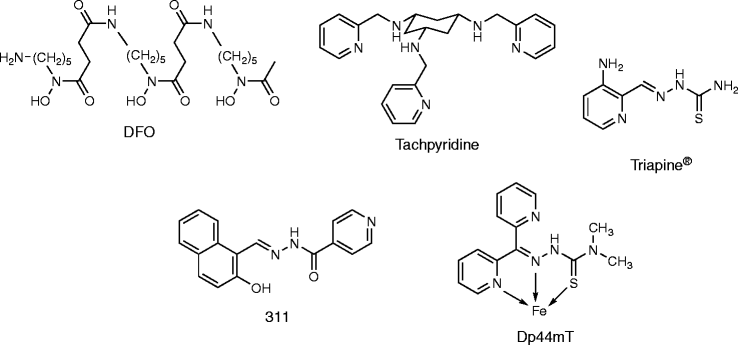Members

5

## Everything posted by Aidan Bradley

1. Ahh I see, and to explain, my only confusion in the beginning was the reaction. I must divide the final precipitate weight by the molar mass of AgCl which will give me both moles of Ag+ and Cl-. From there I can divide the moles of Cl- by 2 since MgCl2 requires 2 Cl- ions to form. So this would then give us the total moles of MgCl2, from which we can divide the weight of the moles by the weight of the original sample, which is 1.0 g. I think this is right, and I will post back whether it works or not. Aha, so it worked. My answer came out to .475 * 100 --> 47.5% of the original sample. Thank you all for the help, I understand this much better now!
2. That makes sense since the Ag+ ions are strong and would force the reaction towards AgCl rather than NaCl
3. Well, from what I can tell, all of them are soluble except when MgCl2 mixes with AgNO3 and NaNO3 to make AgCl and NaCl and Mg(NO3)2, to which AgCl is always insoluble. However, Mg(NO3)2 is also insoluble in many non-polar solvents. I assume they mean water here for the sake of High School AP Chem. So in this case AgCl is our precipitate. Now shall I divide the mass of the precipitate by the molar mass of AgCl? I think that would set me down the path of moles so I could convert into the original MgCl2. But Im second guessing myself because I'm not sure I have the reaction right.
4. So I've been stuck on this problem for about three days or so. It involves precipitation and some reaction, but the reaction is not given: "A 1.0 g mixture of magnesium chloride and sodium nitrate is dissolved in water. Then an excess of silver nitrate solution is added and a precipitate is collected and dried. The mass of the precipitate is 1.43 g. What is the percentage of magnesium chloride in the original mixture?" What I have tried so far is to find out the reaction first, then work backwards to find out how much magnesium chloride reacted with the silver nitrate, but none of the answers I have found match the multiple choice answers listed. I really need to figure this out because I am so lost and confused. -Thanks Aidan B.
×